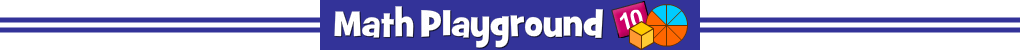Complete the number puzzle using whole numbers.
The number in each block is the sum of the two numbers directly below it.
Number Conundrum Whole Numbers - Learning Connections
Essential Skills
Mental Math - practice number bonds and missing addends

Common Core Connection for Grades 1+
Apply properties of operations as strategies to add and subtract.
Add and subtract within 20, demonstrating fluency for addition and subtraction within 10.
Add within 100, including adding a two-digit number and a one-digit number.
Fluently add and subtract within 20 using mental strategies.
More Math Games to Play